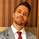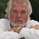2752 lượt xem
I'm pleased to introduce this script in honor of the new array functions introduced to PineScript version 4.0. This update is a long time coming and opens the door to amazing scripting possibilities!

Definition

Named after Charles Spearman and denoted by the Greek letter ‘ρ’ (rho), the Spearman rank correlation coefficient is the nonparametric version of the Pearson correlation coefficient. Use the Spearman rank correlation when you have two ranked variables, and you want to see whether the two variables covary. That is, as one variable increases/decreases, the other variable tends to increase/decrease respectively.

It is best used to discover if two variables (and in this first version of the indicator, two ticker symbols) increase and decrease together. There are advantages to using this version of correlation vs. the Pearson R.

Interpretation

The value oscillates between +1 and -1.

• A value of +1 means the two variables are perfectly correlated, that is they are increasing and decreasing together in perfect harmony.
• A value of -1 means the two variables exhibit a perfect negative correlation, that is they increase and decrease oppositely.
• A value of zero means the two variables are not correlated at all (noise).
Phát hành các Ghi chú:
• Removed the 'lookahead = true' parameter from the security function, not needed.
• Fixed logic of duplicates to be more straightforward.
Phát hành các Ghi chú:
• Fixed issue with hLines.
Phát hành các Ghi chú:
• Updated symbol input to input.symbol.

## Bình luậnThis publication is now featured in our Editors' Picks. In the name of all TradingView traders, thank you for your valuable contribution to the TradingView community, and congrats!
Phản hồithanks for sharing

I have a published study which implements Pearson correlation coefficient, where I attempted to discover correlation between two variables of the same instrument, such as Price(Close) and OBV. What would be you thoughts of such kind of usage?

Cheers
Phản hồidgtrd
@dgtrd, Spearman is superior when all you are looking for is if the two instruments are increasing and decreasing together. Pearson will go a step further to give weight to the magnitudes of increases and decreases.
Phản hồiI love it.
Question: is there a simple way to make it a function? For example, I want to make a correlation "ribbon" with 20 correlations, each a different length:
Plot(correlation(5), color=color.blue)
Plot(correlation(10), color=color.blue)
Plot(correlation(20), color=color.blue)
Plot(correlation(40), color=color.blue)
etc.
I will understand if its not feasible in the current format...but i wanted to ask!
Phản hồi